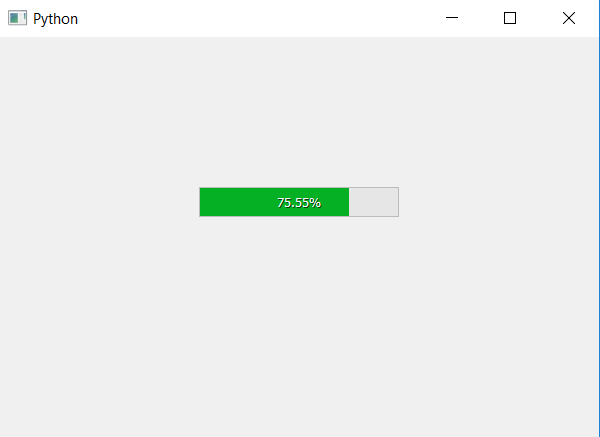Related Articles

# PyQt5 | How to display decimal values in Progress Bar ?

• Last Updated : 22 Apr, 2020

In this article we will see how to display the decimal values in Progress Bar. We can set the value in progress bar using `setValue` method but it takes integer as argument. If we try to pass float or double value, it will automatically convert them into integer.

In order to do this we have to do the following :

1. Change the maximum range value of progress bar, by default it is from 0 to 100. If we want to show 1 decimal value set it to 100*n here ‘n’ will be 10, if we want to show 2 decimal values set it to 100*n here n will be 100 and so on.
2. Set value to progress bar by multiplying it by ‘n’.
3. Use format method to display the decimal value.

Below is the implementation.

 `# importing libraries``from` `PyQt5.QtWidgets ``import` `*` `from` `PyQt5 ``import` `QtCore, QtGui``from` `PyQt5.QtGui ``import` `*` `from` `PyQt5.QtCore ``import` `*` `import` `sys`` ` ` ` `class` `Window(QMainWindow):`` ` ` ` `    ``def` `__init__(``self``):``        ``super``().__init__()`` ` `        ``# setting title``        ``self``.setWindowTitle(``"Python "``)`` ` `        ``# setting geometry``        ``self``.setGeometry(``100``, ``100``, ``600``, ``400``)`` ` `        ``# calling method``        ``self``.UiComponents()`` ` `        ``# showing all the widgets``        ``self``.show()`` ` `    ``# method for widgets``    ``def` `UiComponents(``self``):`` ` `        ``# creating progress bar``        ``bar ``=` `QProgressBar(``self``)`` ` `        ``# setting geometry to progress bar``        ``bar.setGeometry(``200``, ``150``, ``200``, ``30``)`` ` `        ``# setting value of n for 2 decimal values``        ``n ``=` `100`` ` `        ``# setting maximum value for 2 decimal points``        ``bar.setMaximum(``100` `*` `n)`` ` `        ``# value in percentage``        ``value ``=` `75.55`` ` `        ``# setting the value by multiplying it to 100``        ``bar.setValue(value ``*` `n)`` ` `        ``# displaying the decimal value``        ``bar.setFormat(``"%.02f %%"` `%` `value)`` ` `        ``# setting alignment to centre``        ``bar.setAlignment(Qt.AlignCenter)    `` ` ` ` `# create pyqt5 app``App ``=` `QApplication(sys.argv)`` ` `# create the instance of our Window``window ``=` `Window()`` ` `# start the app``sys.exit(App.``exec``())`

Output :Attention geek! Strengthen your foundations with the Python Programming Foundation Course and learn the basics.

To begin with, your interview preparations Enhance your Data Structures concepts with the Python DS Course. And to begin with your Machine Learning Journey, join the Machine Learning – Basic Level Course

My Personal Notes arrow_drop_up